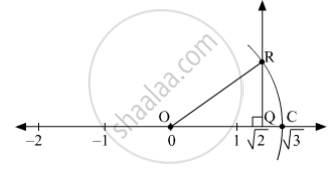# The Number √ 2 is Shown on a Number Line. Steps Are Given to Show √ 3 on the Number Line Using √ 2 . Fill in the Boxes Properly and Complete the Activity. - Mathematics

Sum

The number sqrt2 is shown on a number line. Steps are given to show sqrt3 on the number line using sqrt2. Fill in the boxes properly and complete the activity.

Activity :

• The point Q on the number line shows the number ...... .
• A line perpendicular to the number line is drawn through the point Q. Point R is at unit distance from Q on the line.
• Right angled  ORQ is obtained by drawing seg OR.

l  (OQ) = sqrt2 ,    l(QR) = 1

therefore by Pythagoras theorem,

[l(OR)]^2  = [l(OQ)]^2 + [l(QR)]^2

= _____2 + _____2 = _____ + _____

= ______

∴ l(OR) = _____

Draw an arc with centre O and radius OR. Mark the point of intersection of the line and the arc as C. The point C shows the number √3.

#### Solution•  The point Q on the number line shows the number √2.
• A line perpendicular to the number line is drawn through the point Q. Point R is at unit distance from Q on the line.
• Right angled ∆ ORQ is obtained by drawing seg OR.
• l(OQ) = √2 , l(QR) = 1

∴ by pythagoras theorem,

• [l(OR)]2 = [l(OQ)]2 + [l(QR)]2

= √22 + 12 = 2 + 1

= 3

∴ l(OR) = √3

Draw an arc with centre O and radius OR. Mark the point of intersection of the line and the arc as C. The point C shows the number line √3 .

Concept: Rational Numbers on a Number Line
Is there an error in this question or solution?

#### APPEARS IN

Balbharati Mathematics 8th Standard Maharashtra State Board
Chapter 1 Rational and Irrational numbers
Practice Set 1.4 | Q 1 | Page 5